# Approximating Earth as a sphere

Isaac Newton suggested in 1687 that the earth is not a perfectly round sphere but rather an ellipsoid, and he was right. But since our planet is roughly a sphere, it’s often useful to approximate it by a sphere. So if you’re going to do that, what radius do you use? More generally, what radius do you use when approximating any ellipsoid by a sphere?

This post will discuss the more general problem of finding the radius when approximating any ellipsoid by a sphere. We will give the answer for Earth in particular, and we’ll show how to carry out the calculations. Most of the calculations are easy, but some involve elliptic integrals and we show how to compute these in Python.

## Ellipsoids and spheroids

First of all, what is an ellipsoid? It is a surface whose (x, y, z) coordinates satisfyEarth is an oblate spheroid, which means a = b > c. Specifically, a = b = 6,378,137 meters, and c = 6,356,752 meters.

If you wanted to approximate an ellipsoid by a sphere, you could use

r = (a + b + c)/3.

Why? Because the knee-jerk reaction whenever you need to reduce a set of numbers to one number is to average them.

## Volume of an ellipsoid

We could do a little better, depending on what property of the ellipsoid we’d like to preserve in our approximation. For example, we might want to create a sphere with the same volume as the ellipsoid. In that case we’d use the geometric mean

r = (abc)1/3.

This is because the volume of an ellipsoid is 4πabc/3 and the volume of a sphere is 4πr3/3.

For the particular case of the earth, we’d use

(a2c)1/3 = 6371000.7

## Surface area of an ellipsoid

For some applications we might want a sphere with the same surface area as the ellipsoid rather than the same volume.

The surface area of an ellipsoid is considerably more complicated than the volume. For the special case of an oblate spheroid, like earth, the area is given bywhere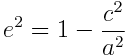The surface area of a sphere is 4 πr2 and so the following code computes r.

    from math import sqrt, atanh
e = sqrt(1 - (c/a)**2)
r = a*sqrt(1 + (1-e**2)*atanh(e)/e) / sqrt(2)


This gives r = 6371007.1 for the earth, about 6.4 meters more than the number we got matching volume rather than area.

For a general ellipsoid, the surface area is given by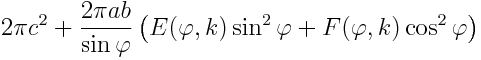where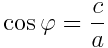and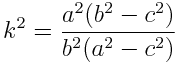Here F is the “incomplete elliptic integral of the first kind” and E is the “incomplete elliptic integral of the second kind.” The names are historical artifacts, but the “elliptic” part of name comes from the fact that these functions were discovered in the context of arc lengths with ellipses, so it shouldn’t be too surprising to see them here.

## Computing ellipsoid surface area in Python

In SciPy, F(φ, k) is given by ellipkinc and E(φ, k) is given by ellipeinc. Both function names start with ellip because they are elliptic functions, and end in inc because they are “incomplete.” In the middle, ellipeinc has an “e” because it computes the mathematical function E(φ, k).

But why does ellipkinc have a “k” in the middle? The “complete” elliptic integral of the first kind is K(k) = F(π/2, k). The “k” in the function name is a reminder that we’re computing the incomplete version of the K function.

Here’s the code for computing the surface area of a general ellipsoid:

    from math import sin, cos, acos, sqrt, pi
from scipy.special import ellipkinc, ellipeinc

def area(a, b, c):
phi = acos(c/a)
k = a*sqrt(b**2 - c**2)/(b*sqrt(a**2 - c**2))
E = ellipeinc(phi, k)
F = ellipkinc(phi, k)
elliptic = E*sin(phi)**2 + F*cos(phi)**2
return 2.0*pi*c**2 + 2*pi*a*b*elliptic/sin(phi)


The differences between the various approximation radii are small for Earth. See my next post on elliptical galaxies where the differences are much larger.

# Poor Mercator

This morning’s xkcd cartoon is “What your favorite map projection says about you.” It’s really funny, but poor Mercator comes off as the most boring projection.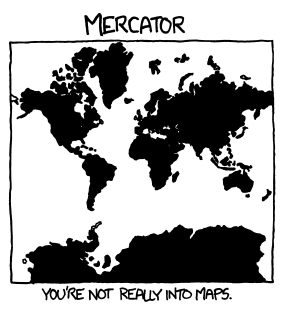Mercator is the most familiar projection, but it has some interesting properties. The most important is that lines of constant bearing on the Earth correspond to straight lines on the map, obviously a desirable property for navigation. More details here.

The inverse of the Mercator projection, going from maps onto the globe, is more interesting. Aside from its geographical importance, it gives a way of relating circular and hyperbolic functions without using complex numbers. More details here.

The Mercator projection is also historically interesting. The modern derivation of the Mercator projection uses logarithms and calculus, but Mercator came up with his projection in 1569 before logarithms or calculus had been discovered. (Update: See more historical detail in Thony C’s comment below.)

# Angle of vertical: geocentric vs astronomical latitude

Don Fredkin left a comment on my previous blog post that surprised me. I found out that latitude doesn’t exactly mean what I thought.

Imagine a line connecting your location with the center of the Earth. I thought that your latitude would be the angle that that line makes with the plane of the equator. And that’s almost true, but not quite.

Instead, you should imagine a line perpendicular to the Earth’s surface at your location and take the angle that that line makes with the plane of the equator.

If the Earth were perfectly spherical, the two lines would be identical and so the two angles would be identical. But since the Earth is an oblate spheroid (i.e. its cross-section is an ellipse) the two are not quite the same.

The angle I had in mind is the geocentric latitude ψ. The angle made by a perpendicular line and the plane of the equator is the geographic latitude φ, also known as the astronomical latitude. The following drawing from Wikipedia illustrates the difference, exaggerating the eccentricity of the ellipse.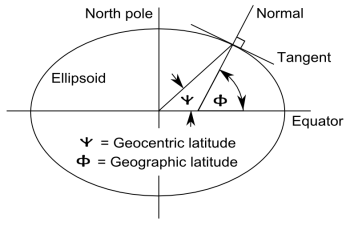How do these two ideas of latitude compare? I’ll sketch a derivation for equations relating geographic latitude φ and geocentric latitude ψ.

Let f(x, y) = (x/a)2 + (y/b)2 where a = 6378.1 km is the equatorial radius and b = 6356.8 km is the polar radius.. The gradient of f is perpendicular to the ellipse given by the level set f(x, y) = 1. At geocentric latitude ψ, y = tan(ψ) x and so the gradient is proportional to (1/a2, tan(ψ) / b2). From taking the dot product with (1, 0) it follows that the cosine of φ is given by

(1 + (a/b)4 tan2 ψ)-1/2.

It follows that

φ = tan-1( (a/b)2 tan ψ )

and

ψ = tan-1( (b/a)2 tan φ ).

The geocentric and geographic latitude are equal at the poles and equator. Between these extremes, geographic latitude is larger than geocentric latitude, but never by more than 0.2 degrees. The maximum difference, as you might guess, is near 45 degrees.

Here’s a graph of φ – ψ in degrees. The difference between these two angles is called the angle of vertical.The maximum occurs at 44.9 degrees and equals 0.1917.

The curve looks very much like a parabola, and indeed it is. The approximation

φ = ψ + 0.186 – 0.0000946667 (ψ – 45)2

is very accurate, within about 0.005 degrees.

Related post: Journey away from the center of the Earth

# Journey away from the center of the Earth

What point on Earth is farthest from its center? Mt. Everest comes to mind, but its summit is the point highest above sea level, not the point farthest from the center. These are not the same because the Earth is not perfectly spherical.

Our planet bulges slightly at the equator due to its rotation. The equatorial diameter is about 0.3% larger than the polar diameter. Sea level at the equator is about 20 kilometers farther from the center of the Earth than sea level at the poles.Photo via Wikipedia

Mt. Everest is about nine kilometers above sea level and located about 28 degrees north of the equator. Chimborazo, the highest point in Ecuador, is seven kilometers above sea level and 1.5 degrees south of the equator.

So how far are Mt. Everest and Chimborazo from the center of the Earth? To answer that, we first need to how far sea level at latitude θ is from the center of the Earth.

Imagine slicing the Earth with a plane containing its polar diameter. To a good approximation (within 100 meters) the resulting shape would be an ellipse. The equation of this ellipse is

(x / a)2 + (y / b)2 = 1

where a = 6378.1 km is the equatorial radius and b = 6356.8 km is the polar radius. A line from the center of the ellipse to a point at latitude θ has equation y = tan(θ) x. Solving the pair of equations for x shows that the distance from the center to the point at latitude θ is

d = sqrt( a2b2 sec2 θ / (a2 tan2 θ + b2 ) )

For Mt. Everest, θ = 27.99 degrees and so d = 6373.4. For Chimborazo, θ = -1.486 degrees and so d = 6378.1. So sea level is 4.7 km higher at Chimborazo. Mt. Everest is 2.6 km taller, but the summit of Chimborazo is about 2.1 km farther away from the center of the Earth.

Update: See my next post for a slight correction. A more accurate calculation would compute sea level is about 4.65 km higher at Chimborazo than Mt. Everest.

# How many trig functions are there?

How many basic trigonometric functions are there? I will present the arguments for 1, 3, 6, and at least 12.

A typical calculator has three trig functions if it has any: sine, cosine, and tangent. The other three that you may see — cosecant, secant, and cotangent — are the reciprocals of sine, cosine, and tangent respectively. Calculator designers expect you to push the cosine key followed by the reciprocal key if you want a secant, for example.

### The calculus textbook answer: 6

The most popular answer to the number of basic trig functions may be six. Unlike calculator designers, calculus textbook authors find the cosecant, secant, and cotangent functions sufficiently useful to justify their inclusion as first-class trig functions.

### The historical answer: At least 12

There are at least six more trigonometric functions that at one time were considered worth naming. These are versine, haversine, coversine, hacoversine, exsecant, and excosecant. All of these can be expressed simply in terms of more familiar trig functions. For example, versine(θ) = 2 sin2(θ/2) = 1 – cos(θ) and exsecant(θ) = sec(θ) – 1.

Why so many functions? One of the primary applications of trigonometry historically was navigation, and certain commonly used navigational formulas are stated most simply in terms of these archaic function names. For example, the law of haversines. Modern readers might ask why not just simplify everything down to sines and cosines. But when you’re calculating by hand using tables, every named function takes appreciable effort to evaluate. If a table simply combines two common operations into one function, it may be worthwhile.

These function names have a simple pattern. The “ha-” prefix means “half,” just as in “ha’penny.” The “ex-” prefix means “subtract 1.” The “co-” prefix means what it always means. (More on that below.) The “ver-” prefix means 1 minus the co-function.

Pointless exercise: How many distinct functions could you come up with using every combination of prefixes? The order of prefixes might matter in some cases but not in others.

The opposite of the historical answer would be the minimalist answer. We don’t need secants, cosecants, and cotangents because they’re just reciprocals of sines, cosines, and tangents. And we don’t even need tangent because tan(θ) = sin(θ)/cos(θ). So we’re down to sine and cosine, but then we don’t really need cosine because cos(θ) = sin(π/2 – θ).

Not many people remember that the “co” in cosine means “complement.” The cosine of an angle θ is the sine of the complementary angle π/2 – θ. The same relationship holds for secant and cosecant, tangent and cotangent, and even versine and coversine.

By the way, understanding this complementary relationship makes calculus rules easier to remember. Let foo(θ) be a function whose derivative is bar(θ). Then the chain rule says that the derivative of foo(π/2 – θ) is -bar(π/2 – θ). In other words, if the derivative of foo is bar, the derivative of cofoo is negative cobar. Substitute your favorite trig function for “foo.” Note also that the “co-” function of a “co-” function is the original function. For example, co-cosine is sine.

### The consultant answer: It depends

The number of trig functions you want to name depends on your application. From a theoretical view point, there’s only one trig function: all trig functions are simple variations on sine. But from a practical view point, it’s worthwhile to create names like tan(θ) for the function sin(θ)/sin(π/2 – θ). And if you’re a navigator crossing an ocean with books of trig tables and no calculator, it’s worthwhile working with haversines etc.

## More trigonometry posts# Inverse Mercator projection

In my earlier post on the Mercator projection, I derived the function h(φ) that maps latitude on the Earth to vertical height on a map. The inverse of this function turns out to hold a few surprises.

The height y corresponding to a positive latitude φ is given by

h(φ) = log( sec(φ) + tan(φ) ).

The inverse function, h-1(y) = φ gives the latitude as a function of height. This function is called the “Gudermannian” after Christoph Gudermann and is abbreviated gd(y). Gudermann was the student of one famous mathematician, Karl Friedrich Gauss, and the teacher of another famous mathematician, Karl Weierstrass.

The Gudermannian function gd(y) can be reduced to familiar functions:

gd(y) = arctan( sinh(y) ) = 2 arctan( ey ) – π/2.

That doesn’t look very promising. But here’s the interesting part: the function gd forms a bridge between hyperbolic trig functions and ordinary trig functions.

sin( gd(x) ) = tanh(x)
tan( gd(x) ) = sinh(x)
cos( gd(x) ) = sech(x)
sec( gd(x) ) = cosh(x)
csc( gd(x) ) = coth(x)
cot( gd(x) ) = csch(x)

By definition, gd(x) is an angle θ whose tangent is sinh(x).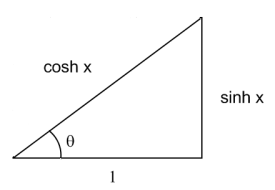In the figure, tan(θ) = sinh(x). Since cosh2(x) – sinh2(x) = 1, the hypotenuse of the triangle is cosh(x). The identities above follow directly from the figure. For example, sin(θ) = sinh(x) / cosh(x) = tanh(x).

Finally, it is easy to show that gd is the inverse of the Mercator scale function h:

h( gd(x) ) = log( sec( gd(x) ) + tan( gd(x) ) ) = log( cosh(x) + sinh(x) ) = log( ex ) = x.

# Mercator projection

A natural approach to mapping the Earth is to imagine a cylinder wrapped around the equator. Points on the Earth are mapped to points on the cylinder. Then split the cylinder so that it lies flat. There are several ways to do this, all known as cylindrical projections.

One way to make a cylindrical projection is to draw a lines from the center of the Earth through each point on the surface. Each point on the surface is then mapped to the place where the line intersects the cylinder. Another approach would be to make horizontal projections, mapping each point on Earth to the closest point on the cylinder. The Mercator projection is yet another approach.With any cylindrical projection parallels, lines of constant latitude, become horizontal lines on the map. Meridians, lines of constant longitude, become vertical lines on the map. Cylindrical projections differ in how the horizontal lines are spaced. Different projections are useful for different purposes. Mercator projection is designed so that lines of constant bearing on the Earth correspond to straight lines on the map. For example, the course of a ship sailing northeast is a straight line on the map. (Any cylindrical projection will represent a due north or due east course as a straight line, but only the Mercator projection represents intermediate bearings as straight lines.) Clearly a navigator would find Mercator’s map indispensable.

Latitude lines become increasingly far apart as you move toward the north or south pole on maps drawn with the Mercator projection. This is because the distances between latitude lines has to change to keep bearing lines straight. Mathematical details follow.

Think of two meridians running around the earth. The distance between these two meridians along a due east line depends on the latitude. The distance is greatest at the equator and becomes zero at the poles. In fact, the distance is proportional to cos(φ) where φ is the latitude. Since meridians correspond to straight lines on a map, east-west distances on the Earth are stretched by a factor of 1/cos(φ) = sec(φ) on the map.

Suppose you have a map that shows the real time position of a ship sailing east at some constant rate. The corresponding rate of change on the map is proportional to sec(φ). In order for lines of constant bearing to be straight on the map, the rate of change should also be proportional to sec(φ) as the ship sails north. That says the spacing between latitude lines has to change according to h(φ) where h‘(φ) = sec(φ). This means that h(φ) is the integral of sec(φ) which equals log |sec(φ) + tan(φ)|. The function h(φ) becomes unbounded as φ approaches ± 90°. This explains why the north and south poles are infinitely far away on a Mercator projection map and why the area of northern countries is exaggerated.

(Update: The inverse of the function h(φ) has some surprising properties. See Inverse Mercator projection.)

The modern explanation of Mercator’s projection uses logarithms and calculus, but Mercator came up with his projection in 1569 before logarithms or calculus had been discovered.

The Mercator projection is now politically incorrect. Although the projection has no political agenda — its design was dictated by navigational requirements — some people are offended that exaggerates the area of northern countries.

For more details of the Mercator projection, see Portraits of the Earth.

# Why care about spherical trig?

Last spring I wrote a post on spherical trigonometry, the study of triangles drawn on a sphere (e.g. the surface of the Earth).Mel Hagen left a comment on that post a few days ago saying

I am revisiting Spherical Trig after 30 years by going back over some of my books that I have collected over the years. …

I asked Mel via email why he was revisiting the subject. He wrote an interesting reply that I am including below with his permission.

Mr. Cook,

Well, let’s see, how did I come to revisit the world of Spherical Trigonometry?

In the early 1970’s I lived within a mile of the coast of the Gulf of Mexico in southern Alabama. I took up daytime sailing with some of my friends from work (they sailed — I just went along for the ride).

Eventually I brought up the concern of being out of sight of land and how do we know where we are. In addition to LORAN navigation receiver and a Radio Direction Finder receiver they always had at least two navigation sextants on board. They demonstrated very quickly and without much detail how to get a “fix.”

I never thought much about it after that until I was at a yard sale in our town and there was a small book, Celestial Navigation for Yachtsmen by Mary Blewitt. I spent the dollar, took it home and spent the next year or so reading it over and over until I had a idea of what was really going on with simple calculations and the examples using tables and charts.

Having taken most of the mathematics courses available in three different colleges I knew there had to be strong basis for Spherical Trigonometry in Celestial Navigation.

Anyway, her book deals exclusively with finding out where you are but not how to point yourself with the true heading for where you want to go. So I dug out several of my old textbooks including the Schaum’s Outline Series by Frank Ayres, Jr., and found just what I was looking for.

Now, let me digress for a moment. In the art of Celestial Navigation you really need three important items: the sextant, a reasonably accurate watch and either a Nautical Almanac or an Air Navigation Almanac. Nowadays you can buy a digital watch for less than \$30.00 that keeps time better than anything they had during World War II. With those 3 items and some simple Spherical Trigonometry you can easily determine your location on the earth (Law of Sines and Law of Cosines).

So, time for a case scenario.

You’re out to sea. A storm comes up. The vessel loses all electronic instrumentation (radios, compasses, computers, LORAN, GPS, etc.).  (If you think this doesn’t happen in real life, think again!) But, as long as you can see the sun during the day you’re in better shape than you think.

Most important — you must have at least one watch that is set for Greenwich Mean Time!

As it is getting about mid-day you start taking sextant sights of the Sun. when it reaches its peak you write down your watch time. With 15 degrees per hour from midnight on your watch you now have local time. With the almanac you can get the declination of the Sun. You should have a reasonable idea of where you think you are. Combine that with two or three Spherical Trigonometry calculations and you can get your “fix.”

Knowing where you are and where you should be headed and using Napier’s Analogies, you can determine where you should point your vessel and get on you way. With a few more calculations you can actually determine how many hours of daylight you have left — and — when the sun will come up in the morning to give you a good reference check.

So curiosity got the best of me. I started playing with Spherical Trigonometry, logarithm tables, and a very good slide rule all over again. The name of the game is not to just use the formulas and the equations but derive them so that you don’t have to try to memorize them and make a mental translation error.

I think one of the things we are doing today is forgetting how we got where we are using certain fields of mathematics and relying too heavily on technology that can easily fail us. Spherical Trigonometry is just an extension of 1 plus 1 equals 2. It just takes some reading and practice, practice, practice.

By the way, another book (if you can find it) that is really helpful is, Standard Mathematical Tables.

Keep in touch. Let me know how people respond to my background and also the sources of information.

Mel Hagen

mbhagen@yahoo.com

# Converting miles to degrees longitude or latitudeSomeone sent me email regarding my online calculator for computing the distance between to locations given their longitude and latitude values. He wants to do sort of the opposite. Starting with the longitude and latitude of one location, he wants to find the longitude and latitude of locations moving north/south or east/west of that location. I like to answer reader questions when I can, so here goes. I’ll give a theoretical derivation followed by some Python code.

Longitude and latitude and are usually measured in degrees, but theoretical calculations are cleaner in radians.  Someone using the Python code below could think in terms of degrees; radians will only be used inside function implementations. We’ll use the fact that on a circle of radius r, an arc of angle θ radians has length rθ. We’ll assume the earth is a perfect sphere. See this post for a discussion of how close the earth is to being a sphere.

## Moving North/South

I’ll start with moving north/south since that’s simpler. Let R be the radius of the earth. An arc of angle φ radians on the surface of the earth has length M = Rφ, so an arc M miles long corresponds to an angle of φ = M/R radians. Moving due north or due south does not change longitude.

## Moving East/West

Moving east/west is a little more complicated. At the equator, the calculation is just like the calculation above, except that longitude changes rather than latitude. But the distance corresponding to one degree of longitude changes with latitude. For example, one degree of longitude along the Arctic Circle doesn’t take you nearly as far as it does at the equator.

Suppose you’re at latitude φ degrees north of the equator. The circumference of a circle at constant latitude φ, a circle parallel to the equator, is cos φ times smaller than the circumference of the equator. So at latitude φ an angle of θ radians describes an arc of length M = R θ cos φ. A distance M miles east or west corresponds to a change in longitude of θ = M/(R cos φ). Moving due east or due west does not change latitude.

## Python code

The derivation above works with angles in radians. Python’s cosine function also works in radians. But longitude and latitude are usually expressed in degrees, so function inputs and outputs are in degrees.

import math

# Distances are measured in miles.
# Longitudes and latitudes are measured in degrees.
# Earth is assumed to be perfectly spherical.

def change_in_latitude(miles):
"Given a distance north, return the change in latitude."

def change_in_longitude(latitude, miles):
"Given a latitude and a distance west, return the change in longitude."
# Find the radius of a circle around the earth at given latitude.
return (miles/r)*radians_to_degrees

# What is the shape of the Earth?

To first approximation, out planet is a sphere. But how accurate is that approximation? What’s a better approximation? How good is that? This post will answer these questions and some related questions.

## How well does a sphere describe the Earth’s shape?

The Earth’s polar diameter is about 43 kilometers shorter than its equatorial diameter, a difference of about 0.3%.This is due to the equatorial bulge caused by the Earth’s rotation.

## What’s a more accurate description of the Earth’s shape?

An oblate spheroid.

## What is an oblate spheroid?

It’s the shape you get by spinning an ellipse around its minor axis. That says if you were to take a cross-section of the Earth containing the polar axis, the shape you get would be an ellipse. The polar axis would be the minor axis and the equatorial axis would be the major axis. But if you were to take a cross-section through the equator, or any plane parallel to the equator, you’d get a circle.

## What is a prolate spheroid?

A prolate spheroid is what you get by spinning an ellipse around its major axis.

## What is an ellipsoid?

An ellipsoid satisfies the following equation.A sphere is an ellipsoid with a = b = c. An oblate spheroid is an ellipsoid with a = b > c. A prolate spheroid is an ellipsoid with a = b < c. A scalene ellipsoid is an ellipsoid for which a, b, and c are all distinct.

## How good is the oblate spheroid model?

The error in approximating the Earth’s shape as an oblate spheroid is less than 100 meters, two orders of magnitude better than the spherical model.

## How are other planets shaped?

The other planets in our solar system are also oblate spheroids with Saturn being the most oblate: the polar diameter of Saturn is about 10% shorter than its equatorial diameter.

## More geodesy posts